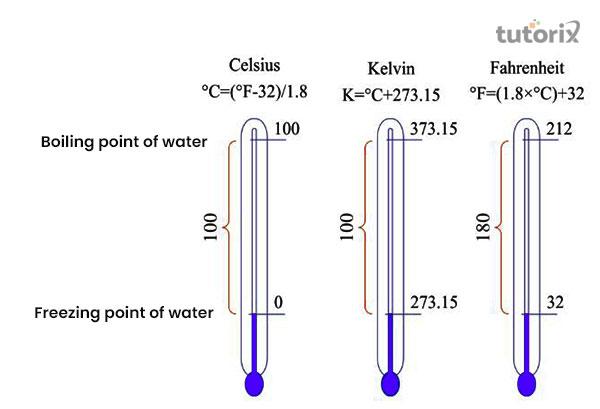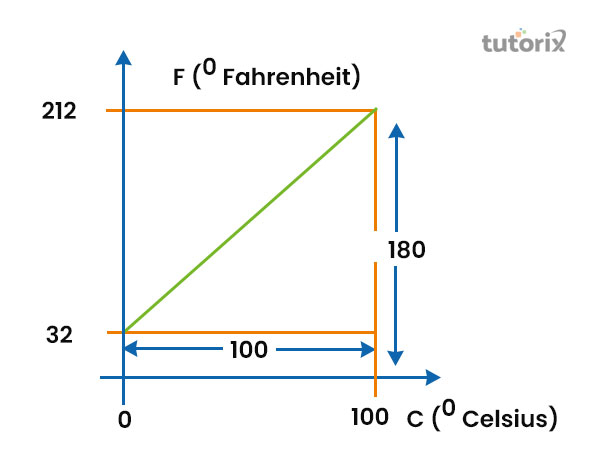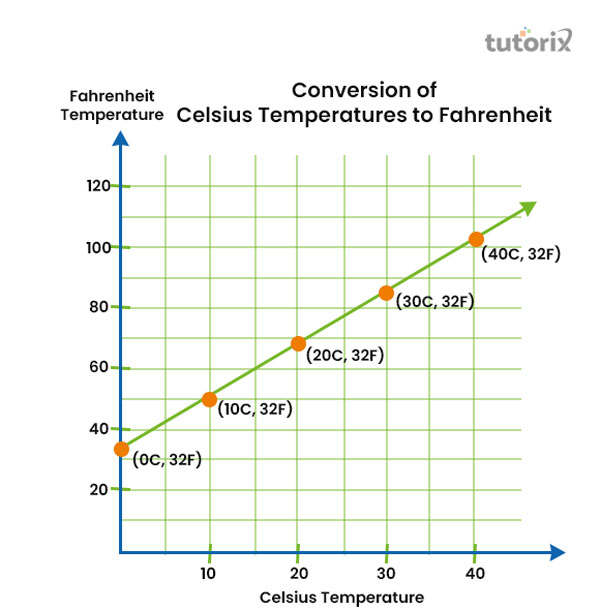# Relation Between Celsius and Fahrenheit

## Introduction

Each Fahrenheit and Celsius scale possesses significant and different temperatures in which the water freezes and boils as well. On a centigrade scale, the temperature is expressed by pointing to degrees Celsius. On another scale, the Fahrenheit scale, the temperature is mainly expressed by pointing to degrees Fahrenheit.

## History and importance of both scales

Between Fahrenheit and Celsius scales, Daniel Gabriel Fahrenheit, a physicist, firstly invented Fahrenheit scale in 1724. This physicist's main concentration was to measure the average inner temperature of a human's body and also to measure the temperature of Brine in which it freezes (Paoletti, 2020).

In the Fahrenheit scale, the Brine, the solution of water , ammonium chloride, and ice freezes at 0°F. As per Fahrenheit's estimation, a human inner body's average temperature is nearly 90°F. This estimation of a person's inner body temperature was later revised as 96°F which was very close to the real inner body temperature.Figure 1: Celsius, Fahrenheit temperature

The centigrade scale was invented in 1742 by Anders Celsius, a Swedish astronomer. The current name, the Celsius scale is derived from the name of this scale's inventor. The Celsius scale was mainly used at the time of its invention as a 100-point system (Sekerák et al. 2020).

This scale was firstly used to measure the difference between the temperature at which the water boils and the snow melts. The word centigrade is derived from the word 'centum' which means 100 and another word 'Gradus' which means steps (Soria-Ruiz, 2021).

According to the Celsius scale, the water freezes at 0°C. The boiling temperature of the water as per the Celsius scale is 100°C. As the difference between these two temperatures is 100 degrees, this scale's another name is Centigrade scale.

## Units of Fahrenheit and Celsius

There are two different units with which the two different temperatures can be identified separately. Every step of the scale is determined with a different unit. Users of the Celsius scale use °C as the unit of temperature according to this scale. Users of the Fahrenheit scale use °F to present temperature, measured by a Fahrenheit scale.Figure 2: Relation between Fahrenheit

## Relation between these two scales

There is a significant relationship between Celsius and Fahrenheit scales as both of these are widely used to measure the temperature of liquid, body, and other materials. The proportional relation within these two scales presents an important fact. In the case of increasing the degree of temperature on a particular scale, the rate of temperature is also found to increase if measured on the other scale (Huda, 2021). As a result, the temperature of the Celsius scales decrease, if the temperature of a thing is found to decrease on the Fahrenheit scale.

Name of the scale Freezing point Boiling point
Celsius 0°C 100°C
Fahrenheit 32°F 212°F

Table 1: Relation between Fahrenheit and Celsius scale

## Conversion of Celsius to Fahrenheit

An important formula can be presented that helps in converting a temperature value from Celsius to Fahrenheit. This conversion process's formula is ''F= (9/5*C) + 32''. In this formula, F is the value of temperature on the Fahrenheit scale and C is the representative of the provided value of temperature on the Celsius scale that has to be converted into Fahrenheit (Yaffe, 2022). As an example, if anyone wants to acquire the Fahrenheit value of 51°C, this formula can be used as follows.

Value of C= 51°C

F = (9/5*51) + 32

F = 123.8°FFigure 3: Celsius temperature to Fahrenheit

The Celsius scale's temperature rises if the temperature of the Fahrenheit scale is also increased. As an example, the temperature is increased from 0°C to 10°C, and as a result the temperature on the Fahrenheit scale also increases from 32°F to 50°F (Hall, 2020). Rather than that 30°C is being increased up to 40°C results in increasing from 86°F to 104°F.

## Conversion of Fahrenheit to Celsius

The formula for converting a Fahrenheit temperature to Celsius is 'C= 5/9 (F-32)'. In this formula as well, F denotes the temperature value of Fahrenheit and C is the representative of Celsius (Sjöberg et al. 2018). As an example, the value of 45°F can be converted into Fahrenheit.

Value of the temperature in Fahrenheit scale: 45°F

C= 5/9 (F-32)

C= 7.22

## Conclusion

All over the world, the Celsius scale is widely used to measure temperature. In future times, the Celsius measurement is cantered to measure the differences in which the water boils versus in which it freezes. During that time, both of these temperatures are placed at the two different ends of a cold and a hot spectrum.

## FAQs

Q1. What is the main base of a Celsius scale?

The freezing and boiling temperature of the water are regarded as the main base for measuring temperature with a Celsius scale. The point of freezing as per this scale is 0°C and at 100°C, the water starts to boil.

Q2. What is the value of 1°C on the Fahrenheit scale?

Based on the formula of converting the Centigrade value to the Fahrenheit scale, 1°C is 33.8°F. This basic value is helpful in calculating other values of this scale.

Q3. What kind of relationship is there between the Celsius and Fahrenheit scale?

A proportional relation can be found within Celsius and Fahrenheit scale. The same changing nature in which the value of a temperature in a scale increases as it increases on the other scale also.

Q4. What are the most three important scales to measure temperature?

The two most important scales are Celsius and Fahrenheit scales. Rather than these two scales, the Kelvin scale is another scale that is also used in recent times to measure temperature.

Q5. Are there any other units of temperature other than Celsius and Fahrenheit?

Other than Celsius and Fahrenheit, Newton, Romer, Rankine, Diesel, and Reaumur are other units. Kelvin is the SI unit, used to measure the temperature.

## References

### Journals

Hall, B. D. (2020, June). Software for calculation with physical quantities. In 2020 IEEE International Workshop on Metrology for Industry 4.0 & IoT (pp. 458-463). IEEE. Retrieved from: https://www.researchgate.net

Huda, N. (2021). Findings of An Equation To Resolve Temperature Conversion Problems All Kinds of Thermometers (Best Practice Discovery Learning Model). In International Seminar On Islamic Education & Peace (Vol. 1, pp. 414-424). Retrieved from: http://ejournal.uniramalang.ac.id

Paoletti, T. (2020). Reasoning about relationships between quantities to reorganize inverse function meanings: The case of Arya. The Journal of Mathematical Behavior, 57, 100741. Retrieved from: https://www.sciencedirect.com

Sekerák, J., Lukáč, S., & Doboš, J. (2020, July). DEVELOPMENT OF INQUIRY SKILLS TO ANALYSE AND DETERMINE RELATIONSHIPS BETWEEN VARIABLES IN MATHEMATICS TEACHING USING GEOGEBRA. In Proceedings of EDULEARN20 Conference (Vol. 6, p. 7th).

Sjöberg, C., Nouri, J., Sjöberg, R., Norén, E., & Zhang, L. (2018, July). Teaching and learning mathematics in primary school trough Scratch. In International Conference on Education and New Learning Technologies, EDULEARN18 Proceedings (pp. 5625-5632). Retrieved on 11th June 2022 from: https://www.researchgate.net

Soria-Ruiz, A. (2021). Value and Scale: Some Observations and a Proposal. Organon F, 28(3), 596-625. Retrieved from: https://run.unl.pt

Yaffe, P. (2022). Workings of science: Can mankind survive scientific illiteracy?. Ubiquity, 2022(February), 1-12. Retrieved from: https://dl.acm.org